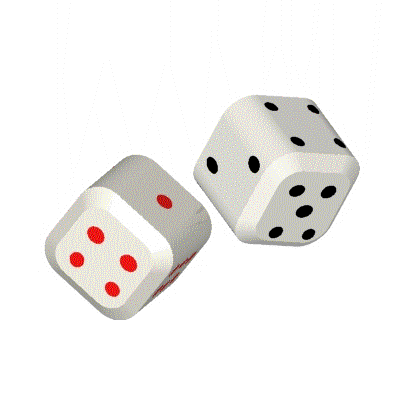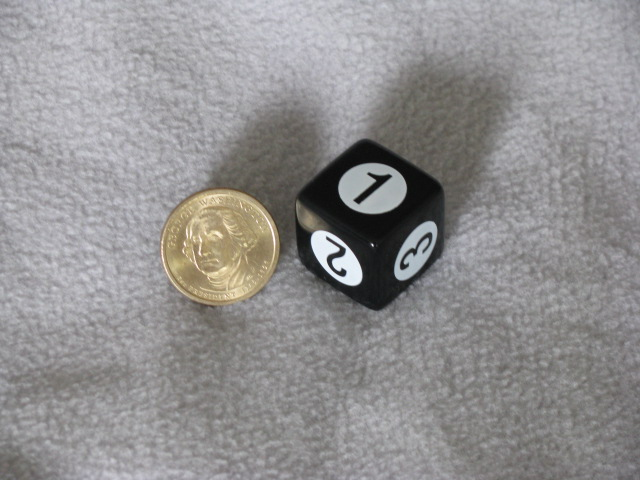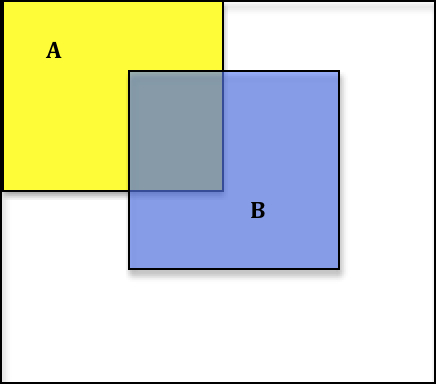# Probabilities: when do we add and when do we multiply?

Posted by: Gary Ernest Davis on: October 27, 2010@davidwees asked on Twitter: “Looking for ideas so students can experiment with the difference between mutually exclusive & independent events in probability.”

My Twitter response was: “May sound strange, but: when do probs add, when do they multiply? Explore.”

In this post I want to think about mutually exclusive and independent events from a teaching point of view.

One issue, as I Tweeted to @davidwees is that we have a definition of mutually exclusive other than probabilities add, but we have no definition of independent other than probabilities multiply (or something immediately equivalent: see the postscript, below).

So let’s look at a simple example where students might explore addition and multiplication of probabilities.

#### Tossing a coin and rolling a die

This example involves tossing a fair coin and rolling a fair die.The elementary events are pairs$(X,n)$ where$X \textrm{ is } H\textrm{ or } T$ and where$n=1, 2, 3, 4, 5 \textrm{ or }6$.

We can lay out these elementary events in a table, as follows:

 (H, 1) (H, 2) (H, 3) (H, 4) (H, 5) (H, 6) (T, 1) (T, 2) (T, 3) (T, 4) (T, 5) (T, 6)

There are 12 elementary events and so$2^{12}=4096$ compound events in total.

Let’s take the event A, consisting of pairs where the die rolls an even number, and the event B where the die rolls an odd number.

So we have:$A=\{(H,2),(T,2),(H,4),(T,4),(H,6),(T,6)\}$

and$B=\{(H,1),(T,1),(H,3),(T,3),(H,5),(T,5)\}$

Is$\textrm{Prob}(A\cup B)=\textrm{Prob}(A)+\textrm{Prob}(B)$ ?

Yes, because$\textrm{Prob}(A)=\frac{6}{12}=\frac{1}{2}, \textrm{Prob}(B)=\frac{6}{12}=\frac{1}{2} \textrm{ and } \textrm{Prob}(A\cup B)=\frac{12}{12}=1$.

Is$\textrm{Prob}(A\cap B)=\textrm{Prob}(A)\times\textrm{Prob}(B)$ ?

No, because$\textrm{Prob}(A\cap B)=\textrm{Prob}(\emptyset)=0 \textrm{ and } \frac{1}{2}\times\frac{1}{2}\neq 0$.

Here are 2 events, both of size 4, chosen at random from the elementary events:$A=\{(H,2),(T,2),(T,3),(T,5)\}$

and$B=\{(H,2),(T,1),(T,4),(T,6)\}$

Is$\textrm{Prob}(A\cup B)=\textrm{Prob}(A)+\textrm{Prob}(B)$ ?

No, because$\textrm{Prob}(A\cup B)=\textrm{Prob}(\{(H,2),(T,1),(T,2),(T,3),(T,4),(T,5),(T,6)\})=\frac{7}{12} \textrm{ while } \textrm{Prob}(A)+\textrm{Prob}(B)=\frac{4}{12}+\frac{4}{12}=\frac{8}{12}=\frac{2}{3}\neq \frac{7}{12}$.

These events were chosen randomly using the folowing Excel commands:

 =IF(RAND()<1/2,”H”,”T”) =INT(RAND()*6)+1 =IF(RAND()<1/2,”H”,”T”) =INT(RAND()*6)+1 =IF(RAND()<1/2,”H”,”T”) =INT(RAND()*6)+1 =IF(RAND()<1/2,”H”,”T”) =INT(RAND()*6)+1

x

The answer of$\frac{7}{12}$ for$\textrm{Prob}(A\cup B)$ is just$\frac{1}{12}$ short of$\textrm{Prob}(A)+\textrm{Prob}(B)=\frac{4}{12}+\frac{4}{12}=\frac{8}{12}$.

That${\frac{1}{12}}^{\textrm{th}}$ comes from the elementary event$(H,2)$ being in both$A \textrm{ and } B$ but only once in$A\cup B$.

This situation is typical: any time$A\textrm{ and } B$ have elementary events in common, those elementary events will only be counted once in$A\cup B$ but will be in both$A\textrm{ and in } B$. In this situation we will have$\textrm{Prob}(A\cup B) < \textrm{Prob}(A)+\textrm{Prob}(B)$.

On the other hand, if$A\cap B = \emptyset$ then the number of – equally likely – events in$A\cup B$ is just the sum of the number of lelmentary events in$A$ and the number of elementary events in$B$.

So, at least for the example above,$\textrm{Prob}(A\cup B)=\textrm{Prob}(A)+\textrm{Prob}(B)$ exactly when$A\cap B =\emptyset$.

#### When do probabilities multiply?

When might we have$\textrm{Prob}(A\cap B)=\textrm{Prob}(A)\times \textrm{Prob}(B)$ ?

In the situation of our example,$\textrm{Prob}(A)=\frac{\textrm{\# elements in A}}{12}$.

So:$\textrm{Prob}(A\cap B)=\frac{\textrm{\# elements in} A\cap B}{12}$

while$\textrm{Prob}(A)\times =\textrm{Prob}(B)=\frac{\textrm{\# elements in A}}{12}\times \frac{\textrm{\# elements in B}}{12}$$= \frac{\textrm{\# elements in A}\times \textrm{\# elements in B}}{144}$.

and we have$\textrm{Prob}(A\cap B)=\textrm{Prob}(A)\times \textrm{Prob}(B)$ exactly when:$12\times \text{\# elements in} A\cap B= \text{\# elements in A}\times \text{\# elements in B}$

An example would be when$\textrm{\# elements in} A\cap B=1$ and$\textrm{\# elements in A}=3, \text{\# elements in A}=4$

For example:$A=\{(H,1),(T,2),(H,3)\}\textrm{ and } B=\{(H,3),(T,4),(H,5),(H,6)\}$.

There are many other pairs of events$A, B \textrm{ with }\textrm{Prob}(A\cap B)=\textrm{Prob}(A)\times \textrm{Prob}(B)$

#### Student exploration

Because “independence” is a name given to events$A, B \textrm{ for which }\textrm{Prob}(A\cap B)=\textrm{Prob}(A)\times \textrm{Prob}(B)$ it seems pedagogically sensible to first encourage students to explore, in simple examples like that above, how it might happen that$\textrm{Prob}(A\cap B)=\textrm{Prob}(A)\times \textrm{Prob}(B)$.

When students have many examples of this phenomenon, it then – and only then – makes sense to give it a name: “independence”.

To first define “independence” in terms of$\textrm{Prob}(A\cap B)=\textrm{Prob}(A)\times \textrm{Prob}(B)$ is to enter the realm of advanced mathematical thinking – a realm where examples conform to abstract definitions, and properties are logical deductions from the definitions. Beginning students need to see definitions as organizing names for an idea, that “begs to be organized” from many examples.

#### Postscript

Many Tweeps, and some commentators, have opined that the basic way of expressing independence is$\textrm{Prob}(A\vert B)=\textrm{Prob}(A)$.

This is fine if one knows what is$\textrm{Prob}(A\vert B)$.

An answer such as “The probability that A happens given that you know B has happened” is not very helpful in actually calculating probabilities.

For that one needs a more precise definition and the usual one is$\textrm{Prob}(A\vert B)=\frac{\textrm{Prob}(A\cap B)}{\textrm{Prob}(B)} \textrm{ provided } \textrm{Prob}(B)>0$.

One can then define independence by$\textrm{Prob}(A\vert B)=\textrm{Prob}(A) \textrm{ when } \textrm{Prob}(B)>0$ but that is exactly equivalent to$\textrm{Prob}(A\cap B)=\textrm{Prob}(A)\times \textrm{Prob}(B)$.

Conditional probabilities are, in my experience, quite difficult for students of all ages to get their heads around.

If someone can suggest a series of explorations that assist high school students to become comfortable working with conditional probability then that would, of course, be another route to independent events.

If you have such explorations, or know of them, I would be very glad to hear of them, as would the mathematics teachers whose questions prompted this post.

Sharing such knowledge about the learning and teaching of mathematics is what the Republic of Mathematics is all about.

An example that illustrates – to me at least – how thorny are both conditional probability and independence for students comes from geometric probability.

Suppose we consider measurable subsets of a unit square$S=[0,1]\times [0,1]$ – sets that have a well-defined area.

Define a probability measure on such sets by area:$\textrm{Prob}(A):=\textrm{area}(A)$.

Now consider the following two sets$A=[\frac{1}{2},1]\times[0,\frac{1}{2}], B=[\frac{1}{4},\frac{3}{4}]\times [\frac{1}{4},\frac{3}{4}]$.Then$\textrm{Prob}(A)=\frac{1}{4}=\textrm{Prob}(B) \textrm{ so} \textrm{Prob}(A)\times \textrm{Prob}(b)=\frac{1}{16}=\textrm{Prob}(A\cap B)$ so$A\textrm{ and } B$ are independent.

Is this any more or less intuitive than$\textrm{Prob}(A\vert B)=\frac{1}{4}=\textrm{Prob}(A)$ ?

### 5 Responses to "Probabilities: when do we add and when do we multiply?"Great point, that the definition is an idea that is “begging to be organized”. I always try to teach that way.

Independent Events, or lack thereof, is a rich topic for kids.
e.g. 3 Door Problem http://en.wikipedia.org/wiki/Monty_Hall_problem; or,
The Lie Detector Problem in which false-negatives overwhelm the false-positives.
These fall under Conditional Probability, yet are certainly accessible to school-age children.Oops, meant to say false-positives overwhelm the true-positives!
e.g. 1000 people tested, 990 negative, 10 positive, only 1 person actually guilty, so P(guilty, given positive test)=1/10 (assuming the guilty tested positive).Independence is often assumed when it is not appropriate. For example: “If there is a 30% chance of rain on Saturday, and a 20% chance of rain on Sunday, then what is the chance that it will rain on both days?” This question is bad, because we know from experience that tomorrow’s weather is likely to resemble today’s weather. On the other hand, events that are widely separated in time or space will usually be independent, or nearly so. I think that it’s a crime to teach the probability formula for independent events without discussing what makes events independent or dependent.Events A and B are independent if the probability of A, given B, is equal to the probability of A. This means that the occurrence or non-occurrence of B gives us no information about A. In symbols, P(A|B) = P(A). For example, if I toss a coin twice, then the outcome of the first toss does not yield any information about the second toss, so the tosses are independent. The equation P(A and B) = P(A)*P(B) is a consequence, but it does not motivate the concept.Thanks for your comments. That’s one way to look at independence, and probably one I prefer, logically. It’s not, however, how everyone introduces independence. Apart from troubles with events with probability 0, the two formulations are equivalent. Also, from a teaching point of view conditional probability is hard. The teaching problem is how to provide a good route for students into these ideas. This is not the same as a logical discussion.